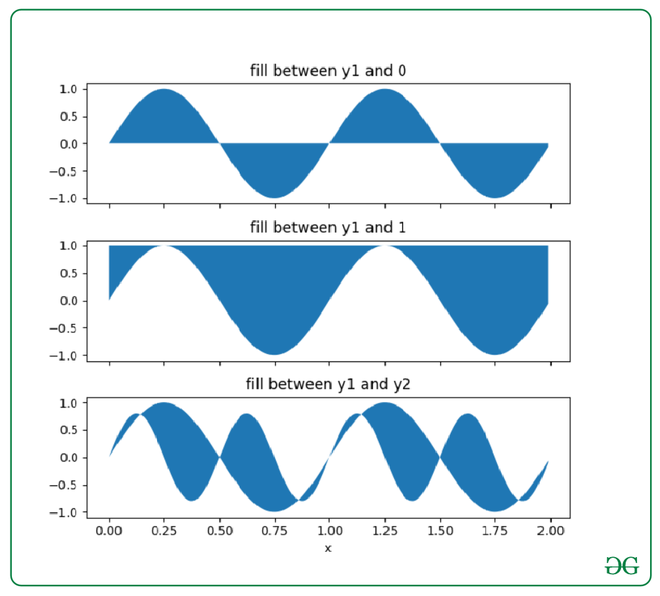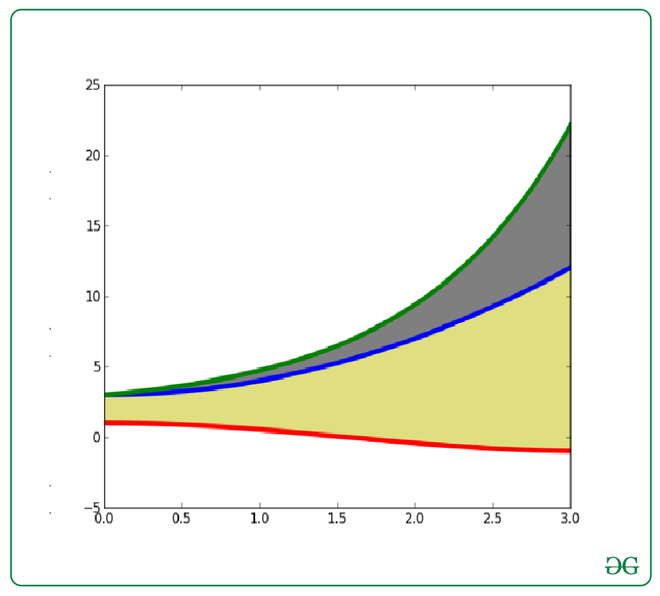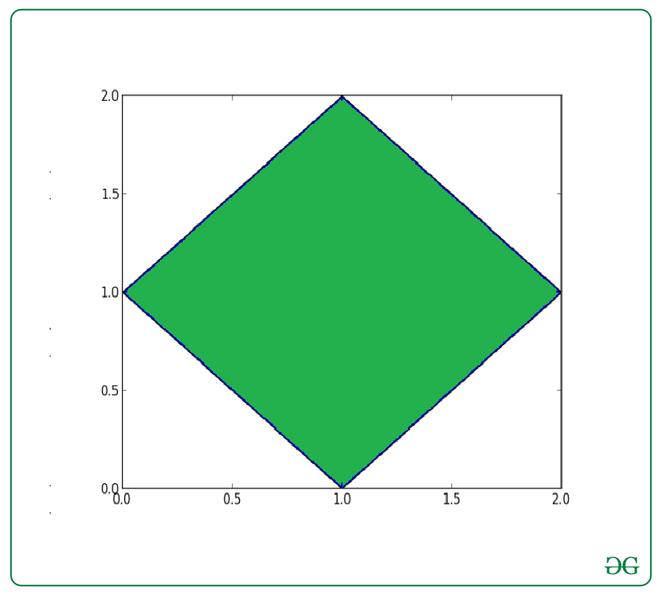# How to Fill Between Multiple Lines in Matplotlib?

• Difficulty Level : Expert
• Last Updated : 08 Oct, 2021

With the use of the fill_between()  function in the Matplotlib library in Python, we can easily fill the color between any multiple lines or any two horizontal curves on a 2D plane.

Syntax: matplotlib.pyplot.fill_between(x, y1, y2=0, where=None, step=None, interpolate=False, *, data=None, **kwargs)

Attention geek! Strengthen your foundations with the Python Programming Foundation Course and learn the basics.

To begin with, your interview preparations Enhance your Data Structures concepts with the Python DS Course. And to begin with your Machine Learning Journey, join the Machine Learning - Basic Level Course

Example 1: Color between the curve of the mathematical function f(x)=sin(x)

## Python3

 `import` `pylab as plt` `import` `numpy as np`   `x ``=` `np.arange(``0.0``, ``2``, ``0.01``)` `y1 ``=` `np.sin(``2` `*` `np.pi ``*` `x)` `y2 ``=` `0.8` `*` `np.sin(``4` `*` `np.pi ``*` `x)`   `fig, (ax1, ax2, ax3) ``=` `plt.subplots(` `  ``3``, ``1``, sharex``=``True``, figsize``=``(``6``, ``6``))`   `ax1.fill_between(x, y1)` `ax1.set_title(``'fill between y1 and 0'``)`   `ax2.fill_between(x, y1, ``1``)` `ax2.set_title(``'fill between y1 and 1'``)`   `ax3.fill_between(x, y1, y2)` `ax3.set_title(``'fill between y1 and y2'``)` `ax3.set_xlabel(``'x'``)` `fig.tight_layout()`

Output:Example 2: Color between the curve of the mathematical function f(x)=cos(x) and f(x)=exp(x) :-

## Python3

 `import` `pylab as plt` `import` `numpy as np`   `X ``=` `np.linspace(``0``, ``3``, ``200``)` `Y1 ``=` `X``*``*``2` `+` `3` `Y2 ``=` `np.sin(X)` `Y3 ``=` `np.cos(X)`   `plt.plot(X, Y1, lw``=``4``)` `plt.plot(X, Y2, lw``=``4``)` `plt.plot(X, Y3, lw``=``4``)`   `plt.fill_between(X, Y1, Y2, color``=``'k'``, alpha``=``.``5``)` `plt.fill_between(X, Y1, Y3, color``=``'y'``, alpha``=``.``5``)`   `plt.show()`

Output:Example 3: Color the Rhombus :-

## Python3

 `import` `matplotlib.pyplot as plt`     `x ``=` `[``1``, ``2``, ``1``, ``0``]` `y ``=` `[``2``, ``1``, ``0``, ``1``]`   `plt.fill(x, y)` `plt.show()`

Output:My Personal Notes arrow_drop_up
Recommended Articles
Page :